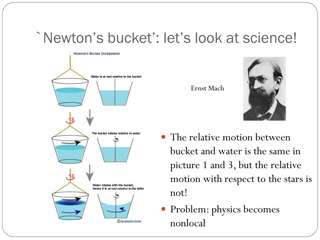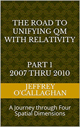# What Newton’s Bucket tell us about space, acceleration and velocity.

Is it an intrinsic property of space that cause velocities to make sense only by saying that this is moving with respect to something while accelerations or changes in velocity don’t require comparisons to give them meaning?

Newton came to conclusion it was based an experiment involving a bucket of water.Greene, Brian describes his book “The Fabric of the Cosmos” (Knopf Doubleday Publishing Group) why he though space
this by observing a spinning of a bucket hanging from rope filled with water.  At first, after it is allowed to unwind the bucket starts to spin but the water inside remains fairly stationary; the surface of the stationary water stays nice and flat. As the bucket picks up speed, little by little its motion is communicated to the water by friction, and the water starts to spin too. As it does, the water’s surface takes on a concave shape, higher at the rim and lower in the center,

“Why does the water’s surface take this shape? Well, because it’s spinning, you say, and just as we feel pressed against the side of a car when it takes a sharp turn, the water gets pressed against the side of the bucket as it spins. And the only place for the pressed water to go is upward. This reasoning is sound, as far as it goes, but it misses the real intent of Newton’s question. He wanted to know what it means to say that the water is spinning: spinning with respect to what? Newton was grappling with the very foundation of motion and was far from ready to accept that accelerated motion such as spinning is somehow beyond the need for external comparisons.

A natural suggestion is to use the bucket itself as the object of reference.  As Newton argued, however, this fails. You see, at first when we let the bucket start to spin, there is definitely relative motion between the bucket and the water, because the water does not immediately move. Even so, the surface of the water stays flat. Then, a little later, when the water is spinning and there isn’t relative motion between the bucket and the water, the surface of the water is concave. So, with the bucket as our object of reference, we get exactly the opposite of what we expect: when there is relative motion, the water’s surface is flat; and when there is no relative motion, the surface is concave.

Newton explained the terrestrial bucket experiment in the following way. At the beginning of the experiment, the bucket is spinning with respect to absolute space, but the water is stationary with respect to absolute space. That is why the water’s surface is flat. As the water catches up with the bucket, it is now spinning with respect to absolute space, and that is why its surface becomes concave. As the bucket slows because of the tightening rope, the water continues to spin spinning with respect to absolute space”and that is why its surface continues to be concave.”

However, Einstein demonstrated, in his Theory of Relativity that absolute space does not exist, therefore their must exist another reason for the concavity of the water in Newton’s
Bucket.

One of the most logical ways to find it would be to use the observable differences between the two types of motion; velocities and accelerations.

For example, velocities transverse the same space or distance in a given time frame while accelerations transverse an exponentially increasing distance over that same time period.

This tells us the primary difference between them is a component of space not time because if one uses the same time frame for both the only thing that distinguishes them is the distance they transverse.

However, Einstein defined the geometry of space and our universe in terms of time therefore, because space not time is, as was just mentioned the variable that distinguishes velocities from accelerations, we should look for a way to define motion purely in terms of its spatial properties.

Einstein gave us the ability to do this when he defined the mathematical relationship between space, time and energy in terms of the constant velocity of light because in doing so, he provided a method of converting a unit of time in a space-time environment to its equivalent unit of space in four *spatial* dimensions.Â  Additionally, because the velocity of light is constant, he also defined a one to one quantitative and qualitative correspondence between his space-time universe and one made up of four *spatial* dimensions.

In other words, Einstein’s mathematics actually defined two mathematically equivalent physical models of the universe, one consisting of four-dimensional space-time and one of only four *spatial* dimensions.

This allows one to define the energy associated with both accelerations and velocities, in terms of a displacement in a “surface” of a three-dimensional space manifold with respect to a fourth *spatial* dimension as well as one in four-dimensional space-time.

In other words, using the spatially equivalent model of Einstein space-time theories one could define energy associated with velocities in terms of a linear displacement in the “surface” of a three-dimensional space manifold with respect to a fourth *spatial* dimension because it remains constant for a given time interval.

While one would define accelerations both gravitational and non gravitational in terms of a non-linear displacement or curvature in that “surface” because, as was mentioned earlier it increases for as it moves through time.

In other words, if one defines gravitational accelerations in terms of a positive non-linear displacement or curvature in that “surface” one would define all other forms of accelerations in terms of an oppositely directed displacement or curvature in that “surface”.

Additionally the magnitude of the these displacements for both accelerations or a those associated with relative velocities is dependent on there energies associated with there movement.

In other words, the greater the relative velocities or accelerations the greater the displacement in the “surface” of the three dimensional space manifold with respect to fourth *spatial *dimension
associated with their motion

What makes accelerated motion different from velocities is that they do not create an energy gradient in space necessary to activate senses because, as was just mentioned the displacement they create on the “surface” of the three dimensional space manifold with respect to a fourth *spatial* dimension is linear.

Therefore, the reason it only makes sense to say that this is moving with respect something is because referencing it to that something provides an energy gradient or differential which can activate measuring equipment or human senses.

However, because Einstein tells us the displacement in the “surface” of a three-dimensional space manifold with respect to fourth *spatial *dimension associated with accelerated motion is non-linear its movement will intrinsically create an energy gradient between two points space which can activate measuring equipment or human senses.

In other words, the reasons it only make sense to say that this is moving with respect something while changes in velocity or accelerations don’t is because acceleration intrinsically cause energy gradients between different points in space where as velocities do not.

This would also explain the observations Newton made in his bucket experiment because the energy or velocity of the water is different at each point in the bucket.  In others word, because the water near the bucket’s edge is moving faster or is accelerated with respect its center it has more energy and therefore will create a larger displacement in the “surface” of a three-dimensional space manifold with respect to a fourth *spatial* dimension resulting in its “surface” becoming a concave.  In other words, the concavity of the surface of the water in Newton bucket is not caused by an interaction with space or the bucket but due to direct effects Einstein showed the energy associated with the velocity of the water has of the geometry of space.

However, this also tells us that what makes space space is energy.

For example, what makes the space in a house and its rooms is not a property of that space but is a property of the geometric structure created by its foundation and walls

Similarly, Einstein tells us that what makes space space in our universe is not a property of space but of the geometric structure created by energy.

Latter Jeff

 The Road to Unifying QM with Relativity part 1 2007 thru 2010Ebook \$8.00Paper Back \$15.00 The Road to Unifying QM with Relativity part 2 2011 thru 2014Ebook \$8.00Paper Back \$16.00 The Road to Unifying QM with Relativity part 3 2015 thru 2020Ebook \$8.00Paper Back \$18.00